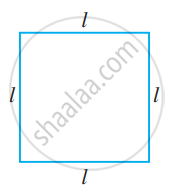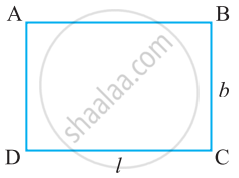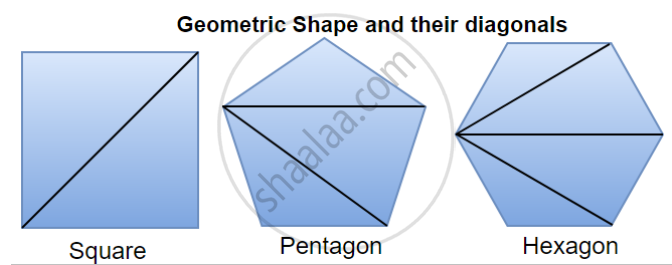# Use of Variables in Common Rules:

## 1. Using Variables To Find The Rule Of Perimeter:

• The perimeter of a square = 4l, "where"  l = the length of the side of the square.
• The perimeter of an equilateral triangle = 3l, "where"  l = length of the side of the equilateral triangle.

• Perimeter of a regular pentagon = 5l, "where"  l = the length of the side of the pentagon.

## 2. Using Variables To Find The Rule Of Area:

• If we denote the length of a square by l, then the area of the square = l2.• If we denote the length of a rectangle by l and its breadth by b, then the area of the rectangle = l × b = lb.• Similarly, If b stands for the base and h for the height of the triangle, then the area of the triangle = 1/2 × b × h.

## 3. Rules for number patterns:

1. If a natural number is denoted by n, its successor is (n + 1). We can check this for any natural number. For example, if n = 10, its successor is n + 1=11, which is known.

2. If a natural number is denoted by n, 2n is an even number and (2n + 1) an odd number. Let us check it for any number, say, 15; 2n = 2 × n = 2 × 15 = 30 is indeed an even number and 2n + 1 = 2 × 15 + 1 = 30 + 1 = 31 is indeed an odd number.

3. The term which occurs at the nth position is given by the expression 3n. You can easily find the term which occurs in the 10th position (which is 3 × 10 = 30); 100th position (which is 3 × 100 = 300) and so on.

## 4. Pattern in geometry:

• Algebraic expressions are used in writing patterns followed by geometrical figures.

• The number of diagonals that we can draw from one vertex of any polygon is (n – 3) where n is the number of sides of the polygon.• Example,
Square = n – 3 = 4 – 3 = 1 diagonal from one vertex.
Pentagon = n – 3 = 5 – 3 = 2 diagonals from one vertex.
Hexagon = n – 3 = 6 – 3 = 3 diagonals from one vertex.

## 5. Rules from arithmetic:

### (i) Commutativity of addition of two numbers:

We know that 4 + 3 = 7 and 3 + 4 = 7
i.e. 4 + 3 = 3 + 4
This property of numbers isknown as the commutativity of addition of numbers.
Let a and b be two variables which can take any number value. Then, a + b = b + a.

### (ii) Commutativity of multiplication of two numbers:

4 × 3 = 12, 3 × 4 = 12
Hence, 4 × 3 = 3 × 4
This property of numbers is known as commutativity of multiplication of numbers.
Let a and b be two variables which can take any number value.
We can express the commutativity of multiplication of two numbers as a × b = b × a

### (iii) Distributivity of numbers:

7 × 38 = 7 × (30 + 8) = 7 × 30 + 7 × 8 = 210 + 56 = 266.
This property is known as distributivity of multiplication over addition of numbers.
Let a, b and c be three variables, each of which can take any number. Then, a × (b + c) = a × b + a × c

### (iv) Associativity Of Addition Of Numbers:

Rule For Associativity Of Addition Of Numbers i.e., (a + b) + c = a + (b + c).

If you would like to contribute notes or other learning material, please submit them using the button below.

### Shaalaa.com

Using Variables To Find The Rule Of Perimeter Of A Square [00:04:55]
S